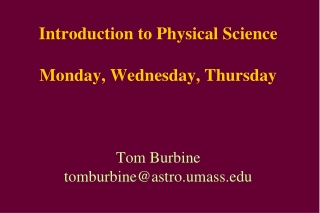DownloadDownload PresentationQuiz tomorrow

# Quiz tomorrow

Download Presentation## Quiz tomorrow

- - - - - - - - - - - - - - - - - - - - - - - - - - - E N D - - - - - - - - - - - - - - - - - - - - - - - - - - -
##### Presentation Transcript

1. Introduction to Physical ScienceMonday, Wednesday, ThursdayTom Burbinetomburbine@astro.umass.edu

2. Quiz tomorrow • Covers everything up to today • I would know: • Order of the planets • speed = distance/time • acceleration = ∆velocity/time • Newton’s three laws of motion • force = mass*acceleration • 1 km = 1,000 meters • 1 kg = 1,000 grams

3. Isaac Newton (1642-1727) • One of the most influential people in human history. • His 1687 publication of the Philosophiæ Naturalis Principia Mathematica (usually called the Principia) is considered to be among the most influential books in the history of science • The book described universal gravitation and the three laws of motion • Newton showed that the motions of objects on Earth and of celestial bodies are governed by the same set of natural laws

4. Isaac Newton • Newton built the first practical reflecting telescope and developed a theory of color based on the observation that a prism decomposes white light into many colors • In mathematics, Newton shares the credit with Gottfried Leibniz for the development of the differential and integral calculus

5. Supposedly saw an apple fall to the ground He then understood that gravity was universal, meaning it affected both the planets and us on Earth Came up with 3 Laws of Motion Isaac Newton

6. Force Force – anything that can cause a body to change velocity

7. Gravity Gravity is a natural phenomenon by which objects with mass attract one another.

8. Newton’s 1st Law In the absence of a net (overall) force acting upon it, an object moves with a constant velocity An object at rest remains at rest An object in motion tends to remain in motion unless a force is acting upon it

9. Why do things on Earth not remain in motion?

10. Newton’s 2nd Law Force = mass x acceleration Units of Force kgm/s2 = newton

11. Newton A Newton is equal to the amount of force required to accelerate a mass of one kilogram at a rate of one meter per second per second

12. How much do you weigh? So much do you weigh Say your mass is 100 kg F = 100 kg x 9.8 m/s2 F = 980 Newtons 9.8 m/s2 is the acceleration of gravity on Earth This is the acceleration due to the Earth’s gravitational field

13. F = ma • F = ma • a = F/m • m = F/a

14. Question • If you apply the same force to two separate carts, one with a mass of 1 kg and one with a mass of 2kg. Which cart will accelerate more?

15. Answer: • If you apply the same force to two separate carts, one with a mass of 1 kg and one with a mass of 2kg. Which cart will accelerate more? • The 1 kg cart will accelerate twice as much

16. Newton’s 3rd Law For any force, there is an equal and opposite reaction force Gravity is holding you on the ground The ground is also pushing back up on you with the same amount of force

17. Action and Reaction • Action: Object A exerts a force on object B • Reaction: Object B exerts a force on object A

18. http://www.vshiksha.com/system/files/u1/pslvc6-rocket.jpg

19. Question: • Which pulls harder, the Moon on Earth, or Earth on the Moon?

20. Answer: • Which pulls harder, the Moon on Earth, or Earth on the Moon? • The same

21. Question: • A bus and a bug have a collision. The force of the bus on the bug splatters all over the windshield. • Is the corresponding force on the bus greater, less, or the same? • Is the resulting deceleration of the bus greater than, less than, or the same as that of the bug?

22. Answer: • A bus and a bug have a collision. The force of the bus on the bug splatters all over the windshield. • Is the corresponding force on the bus greater, less, or the same? • The same • Is the resulting deceleration of the bus greater than, less than, or the same as that of the bug? • Less because the masses are different (a = F/m)

23. Activities • http://www.lth3.k12.il.us/tworivers/Pet%20Projects/Pam%20Winterroth/pam%20winterroth's%20final%20project/Newton's%20laws%20of%20motion%20activities%20page.html

24. Homework